1 Introduction

This is the vignette to explain the implementation of RGCxGC package. This text presents the end to end pipeline for data analysis of comprehensive two dimensional gas chromatography data. You can access a specific function help through the command help([‘function name’]).

This package is designed to analyze data from comprenhensive two-dimensional gas chromatography coupled with mass spctrometry (GCxGC-MS). A general workflow about signal preprocessing in chromatography is summarized in Figure 1. The raw chromatographic signals ussually contains undesirable artifacts, such as chemical and instrumental noise. Therefore, this noise in the data can be significantly reduced by using preprocessing algorithms, herien smoothing, baseline correction, and peak alignment. Then, in order to differentiate between groups/treatments, multivariate analysis is performed.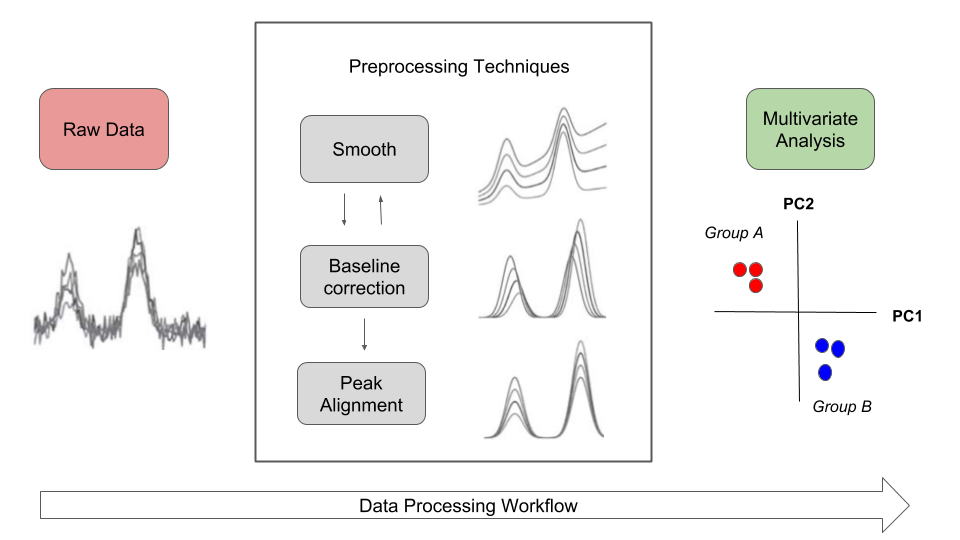Overview of general data processing in chromatography that is presented in the RGCxGC package. The double line between smooth andbaseline correction refers to the interchangeable pathway.

In the RGCxGC package,first, the raw chromatogram is importing from a NetCDF file and is folded into the two-dimensional Total Intensity Chromatogram (2D-TIC).Next, you can perform three preprocessing methodologies in order to remove noise, such as smooth, baseline correction, and peak alignment. Basically, smooth enhance the signal to noise ratio (S/N), baseline correction handles column blooding, and peak alignment corrects the retention time shift of the peaks across multiple runs. Finally, you can perform a multiway principal component analysis (MPCA) to look for systematic patterns that distiguish your samples.

1.1 Basic workflow

The basic workflow of the RGCxGC package is composed of two main steps, preprocessing and multivariate analysis, after the data is imported (Figure 2).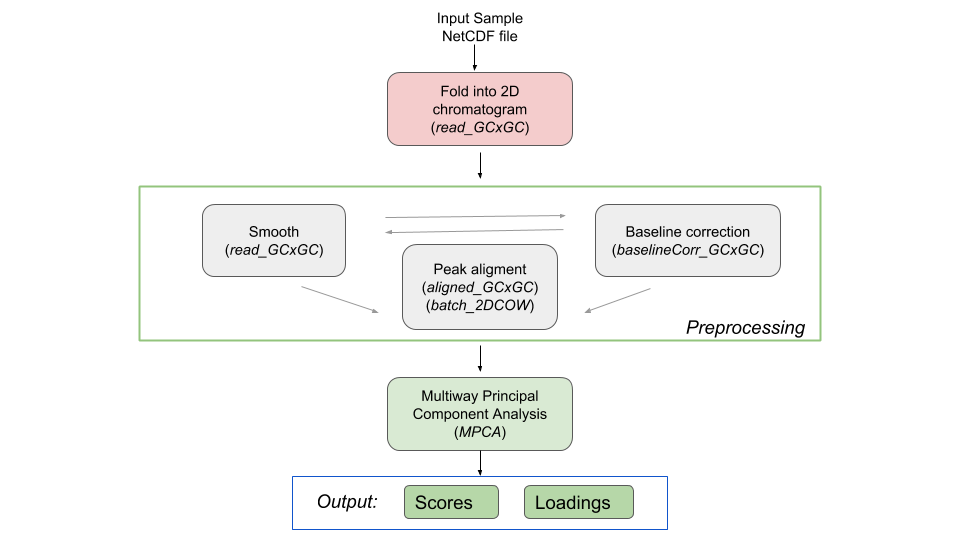The basic workflow of RGCxGC package. The functions for each step are in parenthesis.

The raw NetCDF file is imported with the read_chrom function, in which the user needs to set the modulation time the GCxGC data was acquiered. Next, you can perform smoothing, and baseline correction using the function wsmooth and baseline_corr, respectively. Then, alignment of the peaks from a singel sample, can be done using the function twod_cow based in the two-dimensional correlation optimized warping (2DCOW) algorithm, Alternatively, mutiple sample alignment can be performed with function batch_2DCOW, where the first chromatogram will be cosidered as the reference while aligning the remaining chromatograms. After preprocessing, MPCA can be performed in the dataset using m_prcomp functions, which provide the scores and loadings and the summary, which can be plotted using plot_mpca and scores functions, while the function print you can access to the MPCA summary.

2 Detailed workflow

2.1 Installation

You can install the package by several manners. The most common is to install from CRAN.

install.packages("RGCxGC")

Another way is to install developer version.

library(devtools)
install_github("DanQui97/RGCxGC")

Once you have successfully installed the package, you can acces to every provided functions and data by calling the library.

library(RGCxGC)
##
## Attaching package: 'RGCxGC'
## The following object is masked from 'package:graphics':
##
##     plot
## The following object is masked from 'package:base':
##
##     print

2.2 Importing raw chromatogram

The example data is retrieved form Diagnostic metabolite biomarkers of chronic typhoid carriage. You can access to the whole data with the MetaboLights identifier MTBLS579.

You import the raw chromatogram through the read_chrom function. This function requires at least two parameters: the name of the netCDF file (name), and the modulation time as a integer1. This is an adaptation of Skov and Bro (2008) routine.

chrom_08 <- system.file("extdata", "08GB.cdf", package = "RGCxGC")
MTBLS08 <- read_chrom(chrom_08, mod_time = 5L)
## Warning in base_GCxGC(Object = Object, sam_rate = sam_rate, per_eval =
## per_eval): The last 51 signals will be omitted
## Warning in matrix(tic, nrow = len_1d, ncol = len_2d): la longitud de los
## datos  no es un submúltiplo o múltiplo del número de filas  en
## la matriz
slotNames(MTBLS08)
##  "chromatogram" "time"         "name"         "mod_time"

As one can see, the MTBLS object has four slots. The first correspond to the location of the imported two-dimensional chromatogram, the time slot refers to the retention time, the name slot point to the name of the file, and mod_time to the modulation time.

2.3 Chromatogram visualization

To visualize the chromatogram you can use the plot function. It is built from filled.contour r base function, due to countour plots is a good choice to display non-native GCxGC data (Reichenbach et al., 2004). The default function only plots the most intense signals, into a few breaks. For visualizing a familiar chromatogram, you have to provide the palette color and the number of breaks or levels.

Due to the large variety of relative concentration of metabolites in a sample, the total ion current can also have large variability in the intensity scale. Therefore, you have to set the number of levels in hundreds. As the number of levels increases, you can obtain a more detailed chromatogram. On the other hand, you can use those presented in the colorRamps package (matlab.like & matlab.like2) for the color palette.

# nlevels: Number of levels
# color.palette: The color palette to employ
library(colorRamps)
plot(MTBLS08, nlevels = 100, color.palette = matlab.like)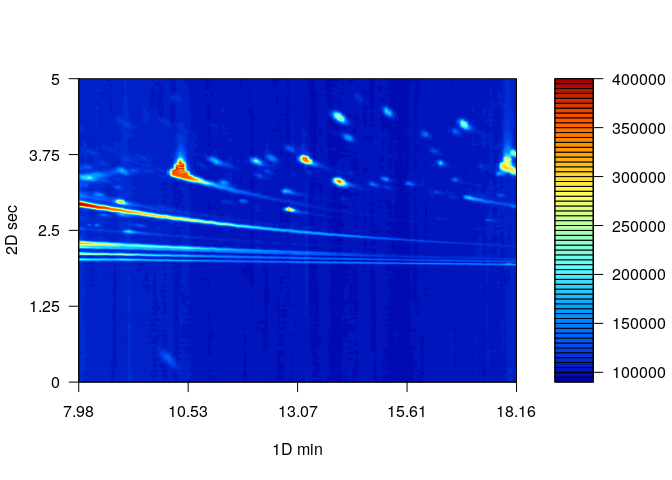2.4 Signal preprocessing

In chromatography, the net metabolite signals are often obscured by instrumental and chemical noise. This undesirable signals variance disturbs the chemical interpretation of the resutls. In order to remove this irrelevant information, some preprocessing steps can be employed. The most common preprocessing algorithms are: baseline correction, smoothing and peak alignment, which are reviewed in the following sections.

2.4.1 Baseline correction

Baseline correction remove a steady increasing intensity in the signal. For example, contamination of the instrument system injection, column bleeding. The baseline correction implemented in this package employs the asymmetric least squares algorithm (Eilers, 2004).

MTBLS08_bc <- baseline_corr(MTBLS08)
plot(MTBLS08_bc, nlevels = 100, color.palette = matlab.like)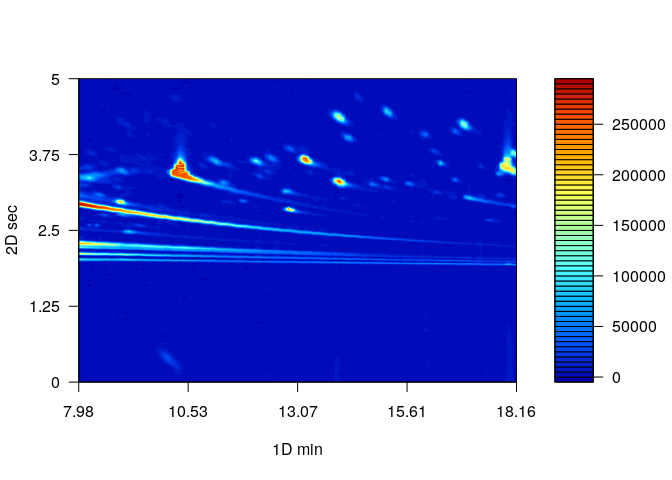The result of this preprocessing step is a cleaner chromatogram. The baseline has been removed and all of the separation space has filled with a more intense blue.

2.4.2 Smoothing

The Whittaker smoother algorithm2 is used in this package (Eilers, 2003). As the main advantages, it accounts by computational efficiency, control over smoothness, automatically interpolation. The Whittaker smoother for GCxGC is implemented in the first chromatogram dimension. In other words, smoothing is carry out to every chromatographic modulation.

The Whittaker smoother works with discrete penalized least squares. For instance, the penalty orde (1 or 2) and the $$\lambda$$ arguments has to be provided. While the penalty refers to the order to penalize the roughness of the signals, $$\lambda$$ is factor which multiply the rougness signal level. Then, greater parameter values will hava a stronger influence of the smmothing.

# Linear penalty with lambda equal to 10
MTBLS08_sm1 <- wsmooth(MTBLS08_bc, penalty = 1, lambda = 1e1)
plot(MTBLS08_sm1, nlevels = 100, color.palette = matlab.like,
main = expression(paste(lambda, "= 10, penalty = 1")) )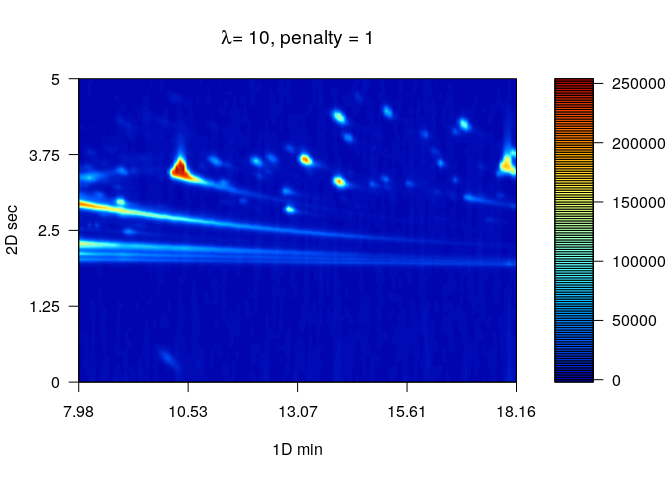# Cuadratic penalty with lambda equal to 10
MTBLS08_sm2 <- wsmooth(MTBLS08_bc, penalty = 2, lambda = 1e1)
plot(MTBLS08_sm2, nlevels = 100, color.palette = matlab.like,
main = expression(paste(lambda, "= 10, penalty = 2")) )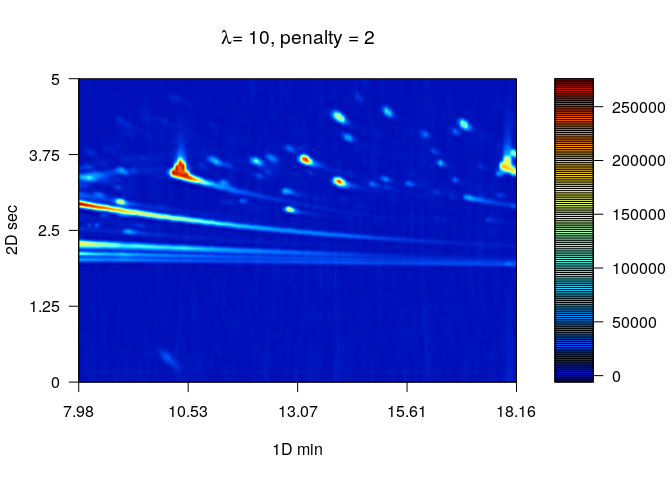2.4.3 Peak alignment

In the chemometric pipeline, the user selects the desired preprocessing steps. However, peak alignment is practically mandatory whenever multiple samples should be compared. The peak alignment corrects unavoidable shifts of the retention times among chromatographic runs.

There are two general pathways for peak alignment: using peak table, or pixel level alignment. In this package, the pixel level alignment is implemented. using the two-dimensional correlation optimized warping (2D-COW) algorithm is implemented (Dabao Zhang et al., 2008). Basically, the algorithm works by splitting the raw chromatogram into $$n$$ segments, then time warping is applied over each dimensions using the one dimensional correlation optimized warping (COW) (Tomasi et al., 2004).

The TwoDCOW need four arguments, the sample, and the reference chromatogram, the number of segments to split the chromatogram and the maximum warping level for both dimensions.

# Raw chromatogram
chrom_09 <- system.file("extdata", "09GB.cdf", package = "RGCxGC")
MTBLS09 <- read_chrom(chrom_09, mod_time = 5L)
## Warning in base_GCxGC(Object = Object, sam_rate = sam_rate, per_eval =
## per_eval): The last 51 signals will be omitted
## Warning in matrix(tic, nrow = len_1d, ncol = len_2d): la longitud de los
## datos  no es un submúltiplo o múltiplo del número de filas  en
## la matriz
# Baseline correction
MTBL09_bc <- baseline_corr(MTBLS09)
# Smoothing
MTBL09_sm2 <- wsmooth(MTBL09_bc, penalty = 2, lambda = 1e1)
# Alignment
aligned <- twod_cow(sample_chrom = MTBLS08_sm2, ref_chrom =  MTBL09_sm2,
segments = c(10, 40), max_warp = c(1, 8))
plot(aligned, nlevels = 100, color.palette = matlab.like,
main = "Aligned chromatogram")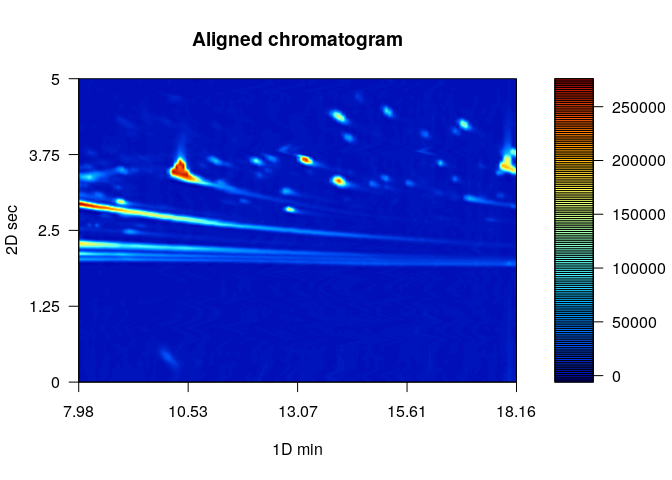2.4.4 Batch peak alignment

Normally, multiple samples are analyzed into batches. Therefore, to align multiple samples simultaneosuly is a more confortable option. The batch_2DCOW function performs this action.

First, you need a vector with the names of the files to be aligned.

chrom_fl <- c(chrom_08, chrom_09)
chrom_fl
##  "/tmp/Rtmpyv8zUA/Rinst335f6ddbbcfd/RGCxGC/extdata/08GB.cdf"
##  "/tmp/Rtmpyv8zUA/Rinst335f6ddbbcfd/RGCxGC/extdata/09GB.cdf"

Then, you must to introduce the same parameters treated above (segments and maximum warping). The default option of batch_2DCOW is to take the first chromatogram as the reference for the alignment.

St_carriege <- batch_2DCOW(chrom_fl, mod_time = 5L,
segments = c(20, 40),
max_warp = c(1, 8))
## Warning in base_GCxGC(Object = Object, sam_rate = sam_rate, per_eval =
## per_eval): The last 51 signals will be omitted
## Warning in matrix(tic, nrow = len_1d, ncol = len_2d): la longitud de los
## datos  no es un submúltiplo o múltiplo del número de filas  en
## la matriz
## Warning in base_GCxGC(Object = Object, sam_rate = sam_rate, per_eval =
## per_eval): The last 51 signals will be omitted
## Warning in matrix(tic, nrow = len_1d, ncol = len_2d): la longitud de los
## datos  no es un submúltiplo o múltiplo del número de filas  en
## la matriz

2.4.5 Get all chromatograms together

Once the chromatograms are already preprocessed, it has to be in a single R object. To meet this requirement, the join_chromatograms joins the chromatograms. Additionally, if you have the metadata, you can also join into the R object. By defautl, the MPCA is carried out with mean centered and scaled data.

In this function, you can provide as many chromatograms or batch chromatograms as you want. What only issue you have to take into consideration is, if you provide a single chromatogram, you have to call the function with a named argument. For example, if you have a chromatogram of name chrom_control, you have to call the function trough (chrom_control = chrom_control).

allChrom <- join_chromatograms(MTBLS09, MTBLS08)

2.5 Multivariate analysis

Multivariate analysis is chosen in the RGCxGC package in order to extract the main metabolites that distinguish the samples. Due to high complexity of the chromatograms containing thousands of variables, the multivariate algorithms is an interesting approach for data analysis in GCxGC.

In this case, the extension of principal component anaylisis is implemente, the called Multiway Principal Component Analysis (MPCA) (Wold et al., 1987). Which can handle the three dimensions of the dataset that is typical for 2D-TIC from GCxGC. of tipical chromatographic experiment.

The example data has to groups, the S. typhy carriage, and the control group. In this sense we are going to perform MPCA with 6 chromatograms.

Names Type
08GB S. typhy Carriege
09GB S. typhy Carriege
14GB S. typhy Carriege
29GB Control
34GB Control
24GB Control

In the previous section, the S. typhy carriage is already aligned. In order to have the same preprocessing techniques for both groups, the control chromatograms will be aligned. This treated data can be called with the name of MTBLS579 name.

data(MTBLS579)

Note: If you would like to work with the whole chromatograms, dowload the MTBLS.rda file from this link.

exp_MPCA <- m_prcomp(MTBLS579)
print(exp_MPCA)
## Importance of components:
##                             PC1      PC2      PC3      PC4      PC5
## Standard deviation     240.1597 42.38155 32.04905 6.29e-13 3.12e-13
## Proportion of Variance   0.9533  0.02969  0.01698 0.00e+00 0.00e+00
## Cumulative Proportion    0.9533  0.98302  1.00000 1.00e+00 1.00e+00
##                              PC6
## Standard deviation     6.514e-14
## Proportion of Variance 0.000e+00
## Cumulative Proportion  1.000e+00

Then, the explained variace of the first principal component is 87% while the explained variance of the second principal component is 5%.

2.5.1 Scores

The scores is the projection in the reduced multivariate space spanned by principal components, and it is related to the (chromatographic) differences among the sampoles. To plot access the scores matrix, you can use the plot_scores function. The second argument is the name of the factor type column to provide colors between groups.

scores(exp_MPCA)
##   Names         PC1        PC2        PC3           PC4           PC5
## 1  08GB -190.775161 -42.114297   7.037531 -6.665708e-13 -2.158370e-13
## 2  09GB -190.775161 -42.114297   7.037531 -6.665708e-13 -2.158370e-13
## 3  14GB -209.195349  52.169556 -44.091279 -6.361299e-13  3.780155e-13
## 4  24GB    4.555358  50.431322  53.150819 -1.879581e-13 -1.254855e-13
## 5  29GB  293.095157  -9.186142 -11.567300  1.062433e-12 -1.413060e-14
## 6  34GB  293.095157  -9.186142 -11.567300  1.062433e-12 -1.413060e-14
##             PC6              Type
## 1 -5.693044e-15 S. typhy Carriege
## 2 -5.693044e-15 S. typhy Carriege
## 3  1.152126e-13 S. typhy Carriege
## 4 -2.655501e-13           Control
## 5  5.550899e-14           Control
## 6  5.550899e-14           Control

While the scores matrix represents the relationship between samples, the loading matrix explain the relationship between variables. Then, we obtain a plot similar to the input chromatograms. To plot loadings, you can use the plot_loading function. The type argument refers to positive (type = “p”) or negative (type = “n”) loading values or (type = “b”) for both loadings values. The default option of this fucntion is to plot the firts principal component, even though, you can choose setting the pc argument.

color.palette = matlab.like)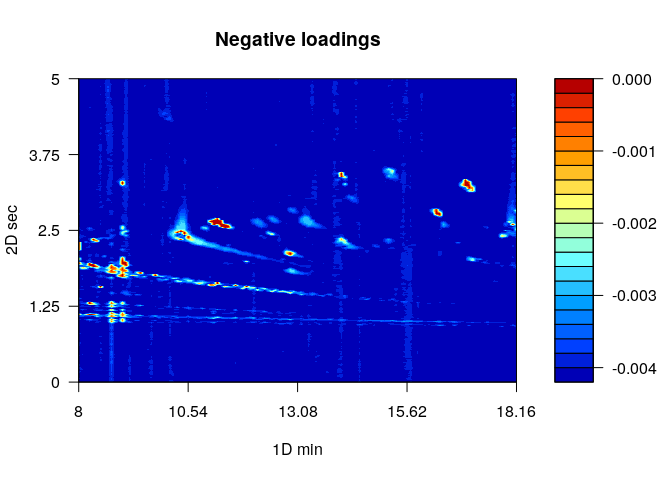color.palette = matlab.like)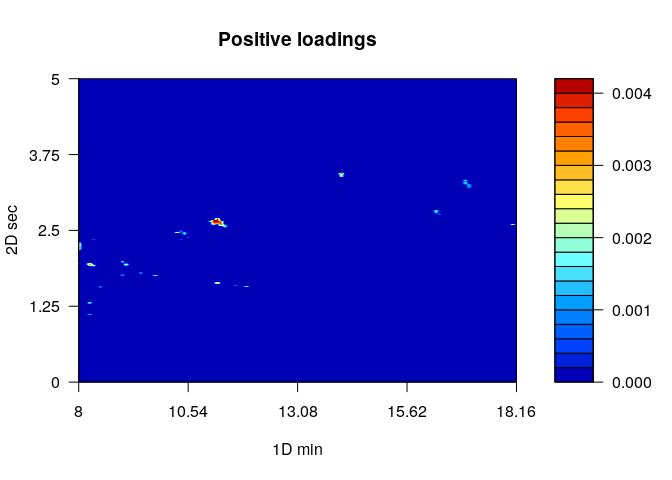# References

Dabao Zhang et al. (2008) Two-dimensional correlation optimized warping algorithm for aligning GCxGC-MS data. Analytical Chemistry, 80, 2664–2671.

Eilers,P.H. (2003) A perfect smoother. Analytical Chemistry, 75, 3631–3636.

Eilers,P.H. (2004) Parametric Time Warping. Analytical Chemistry, 76, 404–411.

Reichenbach,S.E. et al. (2004) Information technologies for comprehensive two-dimensional gas chromatography. Chemometrics and Intelligent Laboratory Systems, 71, 107–120.

Skov,T. and Bro,R. (2008) Solving fundamental problems in chromatographic analysis. Analytical and Bioanalytical Chemistry, 390, 281–285.

Tomasi,G. et al. (2004) Correlation optimized warping and dynamic time warping as preprocessing methods for chromatographic data. Journal of Chemometrics, 18, 231–241.

Wold,S. et al. (1987) Multi-way principal components- and PLS-analysis. Journal of Chemometrics, 1, 41–56.

1. To specify that the modulation time is an integer, an L has to be next of the number.

2. The base functions (with1 & with2) are coupled from the ptw package